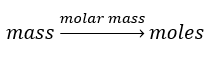# Problem: The vapor pressure of methanol, CH3OH, is 94 torr at 20 °C. The vapor pressure of ethanol, C2H5OH, is 44 torr at the same temperature.(a) Calculate the mole fraction of methanol and of ethanol in a solution of 50.0 g of methanol and 50.0 g of ethanol.

###### FREE Expert Solution

Step 1: Calculate the number of moles of each gas present:Molar mass CH3OH = mass C + mass H + mass O
Molar mass CH3OH = 12 g/mol + (1 g/mol x 4) + (16 g/mol) = 32 g/mol

81% (275 ratings)###### Problem Details

The vapor pressure of methanol, CH3OH, is 94 torr at 20 °C. The vapor pressure of ethanol, C2H5OH, is 44 torr at the same temperature.

(a) Calculate the mole fraction of methanol and of ethanol in a solution of 50.0 g of methanol and 50.0 g of ethanol.